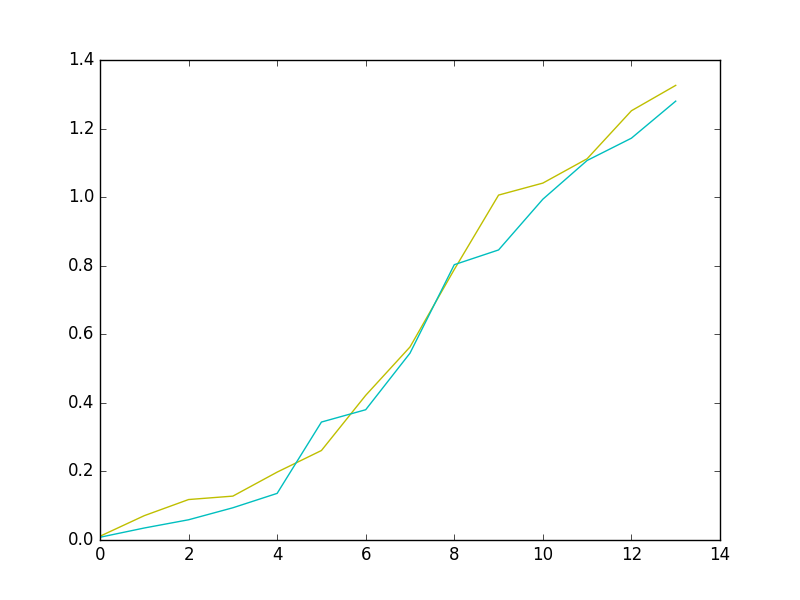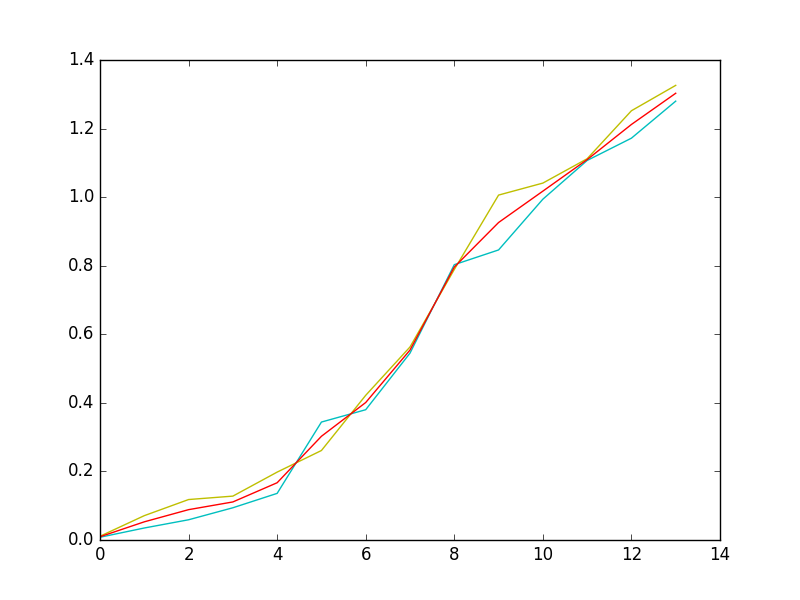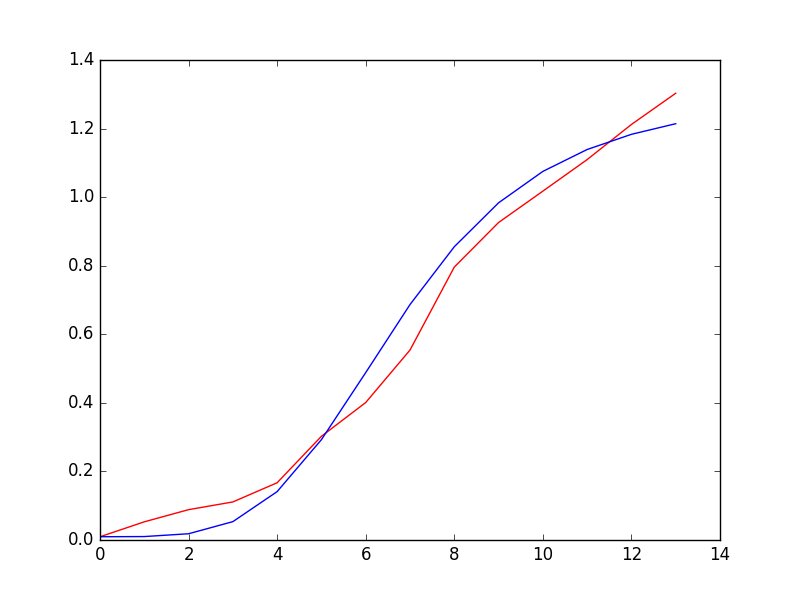# Team:Guanajuato Mx/TheoreticalFit

iGEM Guanajuato Mx

MODEL

# Theoretical fit

#### For the particular case of our project in synthetic biology, we follow the concentration time evolution of P. Aeruginosa obtained through spectrophotometry-UV. Figure 1 shows our experimental results for this measurement, where y-axis is the value of bacteria concentration and the x-axis is the time. We can observe that at short-time the system shows a basal rate of production, after that, the bacteria population increase behaving as exponential function until that finally the system arrive to a stationary state where the bacteria concentration is almost a constant.#### Figure 1. Concentration time evolution of P. Aeruginosa. Experimental results of the concentration of P. Aeruginosa along time.#### Employing a numerical algorithm written in Python, we fitted the experimental points in order to find the best parameters that fit those results, finding that $$K=6.8$$ and $$n=4$$, blue line in figure.